# MA2051 - Ordinary Differential EquationsSample Makeup Exam Solutions - B96

Second Exam - Originally Given 1996 A Term

1
1,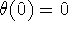,.

Solve the preceding initial-value problem via characteristic equations:. Hence,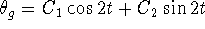;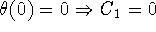;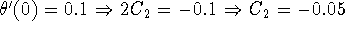. Since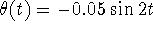, the maximum angular displacement is 0.05 radians.

2
From the preceding solution formula, the period of the pendulum is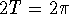or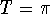s. The pendulum will first return to vertical in half this time (it passes vertical twice in each period) or in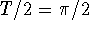s.

Since period is independent of mass, changing the mass will have no effect.

3
Repeating the analysis of part (b) with L in place of 2.45 m shows that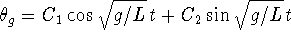. The period is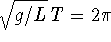or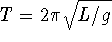. Hence, increasing L increases the period.

4
The characteristic equation ofis. The characteristic roots are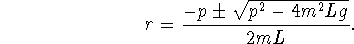Since p is small and both m and L can be made large,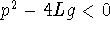; these roots are complex. Their real part is -p/2mL; solutions of this differential equation will be damped by the exponential factor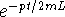. To minimize the effects of this term, choose both m and L as large as possible.

2
Write the second-order equation as the system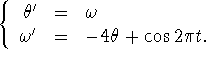Use,,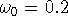. Then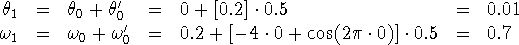3
Characteristic equations:leads to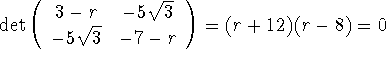. When r = 8, choose p = 1 and find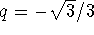. When r = -12, choose p = 1 and find. Hence,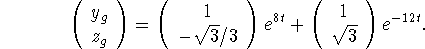4
1
Characteristic equations: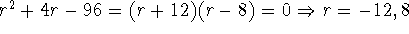: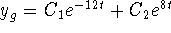.

2
Undetermined coefficients:. Then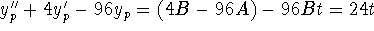forces -96 B = 24 or B = -1/4. Then 4 B - 96 A = 0 yields A = B/24 = -1/96 and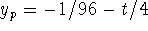. Hence,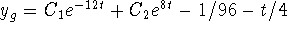.

```

© 1996 by Will Brother.
All rights Reserved. File last modified on December 16, 1996.

```